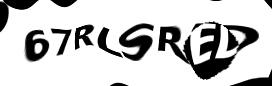There are certain natural numbers that, if you square them and multiply the result by two, yield a number that is one less or one more than a square number. Examples:

• 2·0² = 0 = 1² - 1
• 2·1² = 2 = 1² + 1
• 2·2² = 8 = 3² - 1
• 2·12² = 288 = 17² - 1

Indeed, there are infinitely many of these numbers. I'm asking for a formula to generate them all. For this formula, you may use only constant numbers, the basic arithmetic operations (addition, multiplication, subtraction, division, roots, powers and logarithms) and a variable n. If one substitutes a natural number for n into the formula, the result should be one of the sought numbers. What is the formula? And why does it work?

Remark: I later found out that this number sequence is already known. However, using Google, OEIS or similar to solve the riddle counts as cheating.

Contact and legal info | © 2016-07-31 siquod.org

## Discussion

No entries yetEnter the preceding text: